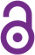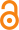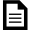# Survival maximization for a Laguerre population

Mario Lefebvre

Article (2002)Preview
Published Version
 Cite this document: Lefebvre, M. (2002). Survival maximization for a Laguerre population. Mathematical Problems in Engineering, 8(6), p. 563-574. doi:10.1080/1024123021000061944

## Abstract

A population whose evolution is approximately described by a Laguerre diffusion process is considered. Let Y(t) be the number of individuals alive at time t and T(y, t(0)(0)() be the first time Y(t) is equal to either 0 or d(> 0), given that Y(t)) = y is in (0, d). The aim is to minimize the expected value of a cost criterion in which the final cost is equal to 0 if Y(T) = d and to if Y(T) = 0. The case when the final cost is 0 (respectively ) if T is greater than or equal to (resp. less than) a fixed constant s is also solved explicitly. In both cases, the risk sensitivity of the optimizer is taken into account.

## Uncontrolled Keywords

Brownian motion; Diffusion processes; Stochastic control; Risk sensitivity; Hitting time; Stochastic differential equation

 Subjects:Open Access document in PolyPublie 2950 Mathématiques appliquées > 2950 Mathématiques appliquées2950 Mathématiques appliquées > 2960 Modélisation mathématique Département de mathématiques et de génie industriel Non applicable CRSNG/NSERC 06 Nov 2018 13:28 07 Nov 2018 01:20 https://publications.polymtl.ca/3379/
 Journal Title:Document issued by the official publisher Mathematical Problems in Engineering (vol. 8, no. 6) Hindawi https://doi.org/10.1080/1024123021000061944